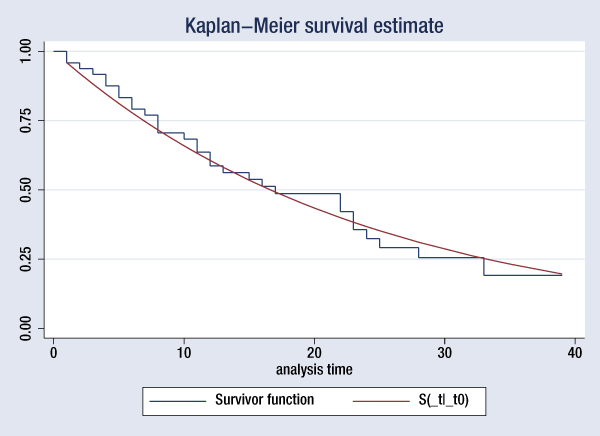»  Home »  Products »  Training »  NetCourses »  NC631: Introduction to survival analysis using StataTraining

## NetCourse® 631: Introduction to survival analysis using Stata

\$295

Discounts available for enrollments of
five or more participants.

Course length: 7 weeks (5 lessons)
Dates: 14 July–1 September 2023
See course schedule.
See alternate dates.

Content:
Learn how to effectively analyze survival data using Stata. We cover censoring, truncation, hazard rates, and survival functions. Topics include data preparation, descriptive statistics, life tables, Kaplan–Meier curves, and semiparametric (Cox) regression and parametric regression. Discover how to set the survival-time characteristics of your dataset just once and then use many of Stata's survival-time estimators and summary statistics commands with those data.

Written for everyone who uses Stata, whether health researchers or social scientists. We provide lesson material, detailed answers to the questions posted at the end of each lesson, and access to a discussion board on which you can post questions for other students and the course leader to answer.

Prerequisites:

• Stata 17 installed and working
• Course content of NetCourse 101 or equivalent knowledge
• Internet web browser, installed and working
(course is platform independent)Isabel Canette
Senior Statistician
StataCorpJoerg Luedicke
Senior Statistician
StataCorpMia Lv
Staff Statistician 2
StataCorp

#### Course content

Lesson 1: Introduction to survival analysis

• Introduction
• The problem of survival analysis
• The need for specific distributions
• Answering specific kinds of questions
• Censoring
• Right-censoring (withdrawal from study)
• Left-censoring
• Truncation
• Left-truncation (delayed entry)
• Right-truncation
• Gaps
• Survival analysis
• The survivor and hazard functions
• Hazard models
• Parametric models
• Semiparametric models
• Nonparametric estimators
• Analysis time (time at risk)
• Summary

Lesson 2: Setting and summarizing survival data

• The purpose of the stset command
• The desired format—Introduction to stset
• The syntax of the stset command
• Specifying analysis time
• Specifying what constitutes failure
• Specifying when subjects exit from the analysis
• Specifying when subjects enter the analysis
• Specifying the subject ID variable
• Handling gaps
• After (st) setting your data
• Look at stset's output
• Use stdescribe
• Use stvary
• Perhaps use stfill
• Example: Hip fracture data
• Appendices
• Dates
• Other formats
• Convenience options

Lesson 3: Nonparametric analysis

• Nonparametric estimation
• The Kaplan–Meier product-limit estimator of the survivor curve
• Calculation of the Kaplan–Meier survivor curve
• Censored observations
• Delayed entry
• Gaps
• Properties of the Kaplan–Meier estimator
• The sts graph command
• The sts list command
• The stsum command
• The Nelson–Aalen estimator of the cumulative hazard
• Alternative estimators of the survivor and cumulative hazard functions
• Comparing survival experience
• The log-rank test
• The Wilcoxon test
• The Tarone–Ware test
• The Peto–Peto–Prentice test
• The Fleming–Harrington test
• Test for trend across ordered groups
• The Cox test

Note: There is a one-week break between the posting of Lessons 3 and 4; however, course leaders are available for discussion.

Lesson 4: Regression models — Cox proportional hazards

• Introduction
• The Cox model has no intercept
• Interpreting coefficients
• The effect of units on coefficients
• The baseline hazard and related functions
• The effect of units on the baseline functions
• Summary of stcox command
• The calculation of results
• No tied failures
• Tied failures
• The marginal calculation
• The partial calculation
• The Breslow approximation
• The Efron approximation
• Summary
• Stratified analysis
• Obtaining coefficient estimates
• Obtaining the baseline functions
• Modeling
• Indicator variables
• Categorical variables
• Continuous variables
• Interactions
• Time-varying variables
• Using stcox with option tvc()
• Using stsplit
• Testing the proportional-hazards assumption
• Tests based on reestimation
• Test based on Schoenfeld residuals
• Graphical methods
• Residuals
• Determining functional form
• Assessing goodness of fit
• Finding outliers and influential points

Lesson 5: Regression models — Parametric survival models

• Introduction
• Classes of parametric models
• Parametric proportional-hazards models
• Accelerated failure-time models
• Maximum likelihood estimation for parametric models
• A survey of parametric regression models in Stata
• Exponential regression
• Exponential regression in the PH formulation
• Exponential regression in the AFT formulation
• Weibull regression
• Weibull regression in the PH formulation
• Weibull regression in the AFT formulation
• Gompertz regression (PH formulation)
• Lognormal regression (AFT formulation)
• Loglogistic regression (AFT formulation)
• Generalized log-gamma regression (AFT formulation)
• Choosing among parametric models
• Nested models
• Nonnested models
• Stratified models
• Use of predict after streg
• Predicting time of failure
• Predicting the hazard and related functions
• Calculating residuals
• Use of stcurve after streg